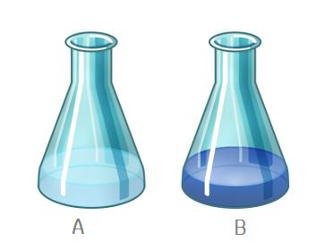The end-point is a physical change in the solution, as determined by the indicator. The end-point is not necessarily the same as the equivalence point, even though both of them represent the same idea (the completion of titration).Figure: Thymolphthalein indicator in a solution. A: good end-point, B: bad end-point.

Example: In sodium hydroxide (NaOH) and hydrochloric acid (HCl), the equivalence point is reached at pH 7. During its titration with thymolphthalein as an indicator, the end-point is reached at pH 9.3.

Read more about equivalence point which often confused with end-point.